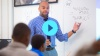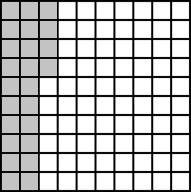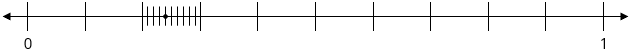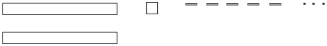# Decimal Fractions

Students expand their concept of what a “number” is as they are introduced to an entirely new category of number, decimals, which they learn to convert, compare, and add in simple cases.

Math

Unit 6

## Unit Summary

This unit introduces 4th grade students to an entirely new category of number—decimals. Students will explore decimals and their relationship to fractions, seeing that tenths and hundredths are particularly important fractional units because they represent an extension of the place value system into a new kind of number called decimals. Thus, students expand their conception of what a “number” is to encompass this entirely new category, which they will rely on for the remainder of their mathematical education.

Students have previously encountered an example of needing to change their understanding of what a number is in 3rd grade, when the term came to include fractions. Their 3rd grade understanding of fractions (3.NF.A), as well as their work with fractions so far this year (4.NF.A, 4.NF.B), will provide the foundation upon which decimal numbers, their equivalence to fractions, their comparison, and their addition will be built. Students also developed an understanding of money in 2nd grade, working with quantities either less than one dollar or whole dollar amounts (2.MD.8). But with the knowledge acquired in this unit, students will be able to work with money represented as decimals, as it so often is.

Thus, students rely on their work with fractions to see the importance of a tenth as a fractional unit as an extension of the place-value system in Topic A, then expand that understanding to hundredths in Topic B. Throughout Topics A and B, students write values in fraction, decimal, unit, and expanded forms to help students understand their equivalence (4.NF.6). Then students learn to compare decimals in Topic C (4.NF.7) and add decimal fractions in Topic D (4.NF.5). Finally, students apply this decimal understanding to solve word problems, including those particularly related to money, at the end of the unit. Thus, the work with money (4.MD.2) supports the major work and main focus of the unit on decimals.

While students will have ample opportunities to engage with the standards for mathematical practice, they’ll rely heavily on looking for and making use of structure (MP.7), particularly the structure of the place value system. They will also construct viable arguments and critique the reasoning of others (MP.3) using various visual models to support their reasoning.

In 5th Grade Math, students will build on this solid foundation of decimal fractions to generalize their understanding of the “ten times as much” relationship in the place value system to include decimals, then to perform decimal operations using strategies based on place value (5.NBT.1—4, 5.NBT.7). Students fluently operate on decimals using the standard algorithm for each operation in 6th Grade Math (6.NS.3). From that point forward, students will use their understanding of decimals as a specific kind of number in their mathematical work, including ratios, functions, and many others.

Pacing: 15 instructional days (13 lessons, 1 flex day, 1 assessment day)

Fishtank Plus for Math

Unlock features to optimize your prep time, plan engaging lessons, and monitor student progress.## Assessment

The following assessments accompany Unit 6.

### Pre-Unit

Have students complete the Pre-Unit Assessment and Pre-Unit Student Self-Assessment before starting the unit. Use the Pre-Unit Assessment Analysis Guide to identify gaps in foundational understanding and map out a plan for learning acceleration throughout the unit.

### Mid-Unit

Have students complete the Mid-Unit Assessment after lesson 8.

### Post-Unit

Use the resources below to assess student understanding of the unit content and action plan for future units.

Expanded Assessment Package

Use student data to drive your planning with an expanded suite of unit assessments to help gauge students’ facility with foundational skills and concepts, as well as their progress with unit content.

## Unit Prep

### Intellectual Prep

#### Intellectual Prep for All Units

• Read and annotate “Unit Summary” and “Essential Understandings” portion of the unit plan.
• Do all the Target Tasks and annotate them with the “Unit Summary” and “Essential Understandings” in mind.
• Take the Post-Unit Assessment.

#### Unit-Specific Intellectual Prep

 Area model Example: The following shape represents 1 whole. 0.24 of it is shaded.Number line Example: The point on the number line below is located at 0.24.Pictorial base ten blocks Example: Represent 21.53 with base ten blocks.### Essential Understandings

• The fractional units tenths and hundredths are particularly important, as they represent the extension of the place value system to places smaller than one whole.
• “There are several ways to read decimals aloud. For example, 0.15 can be read aloud as ‘1 tenth and 5 hundredths’ or ’15 hundredths,’ just as 1,500 is sometimes read ’15 hundred’ or ‘1 thousand, 5 hundred.’ (Mathematicians and scientists often read 0.15 aloud as ‘zero point one five’ or ‘point one five’.)” (NF Progression, p. 15). Understanding the equivalence of these ways of reading a decimal aloud, for example 0.65 as “sixty-five hundredths” or “6 tenths and 5 hundredths” depends on understanding the equivalence of fractions and decimals as well as the equivalence of $$\frac{6}{10} + \frac{5}{100}$$ and $$\frac{65}{100}$$.
• “The number of digits to the right of the decimal point indicates the number of zeros in the denominator, so that $$2.70=\frac{270}{100}$$ and $$2.7=\frac{27}{10}$$” (NF Progression, p. 15).
• Unlike with whole numbers, adding zeros to the end of a decimal number does not change its value. Fraction equivalence can be used to show $$2.70=\frac{270}{100}=\frac{10\times27}{10\times10}=\frac{27}{10}=2.7$$.
• Place-value units are not symmetric about the decimal point; rather, they are symmetric around the ones place.
• Comparing numbers written in standard form uses the understanding that one of any unit is greater than any amount of a smaller unit. Thus, the largest place values in each number contains the most relevant information when comparing numbers. If both numbers have the same number of largest units, the next largest place value should be attended to next, iteratively, until one digit is larger than another in the same unit. This may mean that a decimal with fewer digits is larger than a decimal with more digits in cases where the larger decimal place values have a larger value (e.g., 0.4 > 0.13).
• The approach to adding decimal fractions is based in the idea of needing to add like units together and, thus, replacing decimals with equivalent fractions that allow for addition of like units (i.e., common denominators).

### Materials

• Base ten blocks (1 thousand, 10 hundreds, 10 tens, 10 ones per student or small group) — You could just use one set for the teacher if materials are limited.

### Vocabulary

decimal expanded form

decimal number

decimal point

decimal fraction

fraction expanded form

hundredth

tenth

To see all the vocabulary for Unit 6, view our 4th Grade Vocabulary Glossary.

## Unit Practice

Word Problems and Fluency Activities

Access daily word problem practice and our content-aligned fluency activities created to help students strengthen their application and fluency skills.## Lesson Map

Topic A: Understanding Tenths

Topic B: Understanding Tenths and Hundredths

Topic C: Decimal Comparison

Topic E: Money as a Decimal Amount

## Common Core Standards

Key

Major Cluster

Supporting Cluster

### Core Standards

#### Measurement and Data

• 4.MD.A.2 — Use the four operations to solve word problems involving distances, intervals of time, liquid volumes, masses of objects, and money, including problems involving simple fractions or decimals, and problems that require expressing measurements given in a larger unit in terms of a smaller unit. Represent measurement quantities using diagrams such as number line diagrams that feature a measurement scale.

#### Number and Operations—Fractions

• 4.NF.C.5 — Express a fraction with denominator 10 as an equivalent fraction with denominator 100, and use this technique to add two fractions with respective denominators 10 and 100. Students who can generate equivalent fractions can develop strategies for adding fractions with unlike denominators in general. But addition and subtraction with unlike denominators in general is not a requirement at this grade. For example, express 3/10 as 30/100, and add 3/10 + 4/100 = 34/100.
• 4.NF.C.6 — Use decimal notation for fractions with denominators 10 or 100. For example, rewrite 0.62 as 62/100; describe a length as 0.62 meters; locate 0.62 on a number line diagram.
• 4.NF.C.7 — Compare two decimals to hundredths by reasoning about their size. Recognize that comparisons are valid only when the two decimals refer to the same whole. Record the results of comparisons with the symbols >, =, or <, and justify the conclusions, e.g., by using a visual model.

• 2.MD.C.8

• 4.NBT.A.2

• 3.NF.A.2
• 4.NF.A.1
• 4.NF.A.2
• 4.NF.B.3

• 5.NBT.A.1
• 5.NBT.A.2
• 5.NBT.A.3
• 5.NBT.A.4
• 5.NBT.B.7

• 5.NF.A.1

### Standards for Mathematical Practice

• CCSS.MATH.PRACTICE.MP1 — Make sense of problems and persevere in solving them.

• CCSS.MATH.PRACTICE.MP2 — Reason abstractly and quantitatively.

• CCSS.MATH.PRACTICE.MP3 — Construct viable arguments and critique the reasoning of others.

• CCSS.MATH.PRACTICE.MP4 — Model with mathematics.

• CCSS.MATH.PRACTICE.MP5 — Use appropriate tools strategically.

• CCSS.MATH.PRACTICE.MP6 — Attend to precision.

• CCSS.MATH.PRACTICE.MP7 — Look for and make use of structure.

• CCSS.MATH.PRACTICE.MP8 — Look for and express regularity in repeated reasoning.

Unit 5

Fraction Operations

Unit 7

Unit Conversions

## Request a Demo

See all of the features of Fishtank in action and begin the conversation about adoption.

Yes

No

### Any other information you would like to provide about your school?